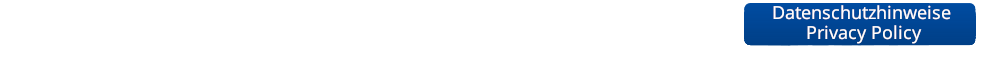Advanced Machine Learning

 Semester: WS 2015 Type: Lecture Lecturer: Credits: V3 + Ü1 (6 ECTS credits)
Course Dates:
Type
Date
Room
Lecture Mon 14:15-15:45 UMIC 025
Lecture/Exercise Thu 10:15-11:45 UMIC 025

## Lecture Description

This lecture will extend the scope of the "Machine Learning" lecture with additional and, in parts, more advanced concepts. In particular, the lecture will cover the following areas:

• Regression techniques (linear regression, ridge regression, lasso, support vector regression)
• Gaussian Processes
• Learning with latent variables
• Dirichlet Processes
• Structured output learning

## Prerequisites

Successful completion of the class "Machine Learning" is recommended, but not a hard prerequisite.

## Exercises

The class is accompanied by exercises that will allow you to collect hands-on experience with the algorithms introduced in the lecture. There will be both pen&paper exercises and practical programming exercises based on Matlab (roughly 1 exercise sheet every 2 weeks). Please turn in your solutions to the exercises by e-mail to the appropriate TA the night before the exercise class.

We ask you to work in teams of 2-3 students.

Course Schedule
Date Title Content Material
Introduction Introduction, Polynomial Fitting, Least-Squares Regression, Overfitting, Regularization, Ridge Regression
Exercise 0 Intro Matlab
Linear Regression I Probabilistic View of Regression, Maximum Likelihood, MAP, Bayesian Curve Fitting
Linear Regression II Basis Functions, Sequential Learning, Multiple Outputs, Regularization, Lasso, Bias-Variance Decomposition
Gaussian Processes I Kernels, Kernel Ridge Regression, Gaussian Processes, Predictions with noisy observations
Gaussian Processes II Influence of hyperparameters, Bayesian Model Selection
Exercise 1 Regression, Least-Squares, Ridge, Kernel Ridge, Gaussian Processes
Probability Distributions Bayesian Estimation Revisited, Conjugate Priors, Bernoulli, Binomial, Beta, Multinomial, Dirichlet, Gaussian, Gamma, Student's t
Approximate Inference I Sampling Approaches, Monte Carlo Methods, Transformation Methods, Ancestral Sampling, Rejection Sampling, Importance Sampling
Approximate Inference II Markov Chain Monte Carlo, Metropolis-Hastings Algorithm, Gibbs Sampling
Mixture Models I Mixtures of Gaussians, ML estimation, EM, latent variables, General EM
Mixture Models II General EM, Mixtures of Bernoulli distributions, Monte Carlo EM, Dirichlet priors, Infinite Mixture Models, Gibbs Sampling
Linear Discriminants Revisited Generalized Linear Discriminants, Gradient Descent, Logistic Regression, Softmax Regression, Error function analysis
Exercise 2 Rejection Sampling, Importance Sampling, Metropolis-Hastings, EM, Mixtures of Bernoulli distributions
Neural Networks Single-Layer Perceptron, Multi-Layer Perceptron, Mapping to Linear Discriminants, Error Functions, Regularization
Backpropagation Multi-layer networks, Chain rule, gradient descent, implementation aspects
Tricks of the Trade Stochastic Gradient Descent, Minibatch Learning, Optimizers (Momentum, Nesterov-Momentum, AdaGrad, RMS-Prop, Ada-Delta, Adam), Drop-out, Batch Normalization
Exercise 3 Softmax, Backpropagation, ...
Exercise 4 Introduction to Theano and Torch7
Convolutional Neural Networks I CNNs, Convolutional layer, pooling layer, LeNet, ImageNet challenge, AlexNet
Convolutional Neural Networks II VGGNet, GoogLeNet, Visualizing CNNs
Dealing with Discrete Data Word Embeddings, word2vec, Motivation for RNNs
Recurrent Neural Networks I Plain RNNs, Backpropagation through Time, Practical Issues, Initialization
Exercise 5 Solution to backprop. exercise, Weight sharing, RNNs.
Recurrent Neural Networks II LSTM, GRU, Success Stories
Unsupervised Learning Restricted Boltzmann Machines
Wrap-up Deep Belief Networks, Glorot Initialization, Reinforcement Learning
Repetition Repetition